## Proof Data Revival Formula

I write again equation (5) for your convenient: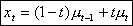(5)

We can rearrange equation (5) to give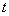: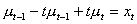, or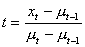(a)

Preparing for other terms: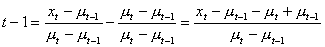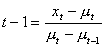(b)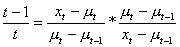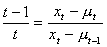(c)

I write again equation (4) in here: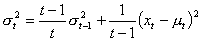(4)

Putting equation (b) and (c) into equation (4) gives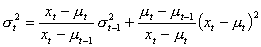(d)

We want to get the value of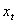from equation (d). For this purpose we arrange equation (d) so that it will give a quadratic equation in.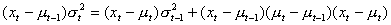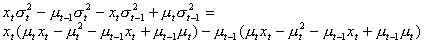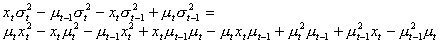Rearrange for: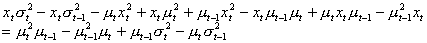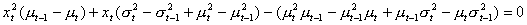(e)

The discriminant of the quadratic equation is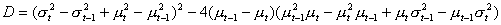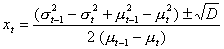(proved)

Preferable reference for this tutorial is

Teknomo, Kardi. (2006) Recursive Average and Variance.
http://people.revoledu.com/kardi/tutorial/RecursiveStatistic/index.html